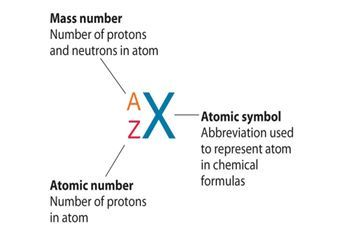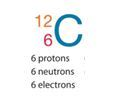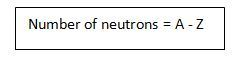# Atomic Number and Mass Number

Atomic number and mass number are always whole numbers because they are obtained by counting whole objects (protons, neutrons, and electrons). The sum of the mass number and the atomic number for an atom (A-Z) corresponds to the total number of subatomic particles present in the atom. The mass number reports the mass of the atom’s nucleus in atomic mass units (amu).

The modern periodic table is arranged in such a way that all the elements have an increasing atomic number, and subsequently, increasing mass number. But do you know what mass number, or even what atomic number is?

Well, as you know, an atom consists of electrons, protons and neutrons. The number of electrons in the outer most shell gives us the valency of the atom. Similarly, the number of protons and neutrons are associated with the atomic number and mass number of the atom.

## Recommended VideosLet’s look at the difference between the two terms.

## What is Atomic Number?

• The total number of protons in the nucleus of an atom gives us the atomic number of that atom.
• It is represented with the letter ‘Z.’
• All the atoms of a particular element have the same number of protons, and hence the same atomic number.
• Atoms of different elements have different atomic numbers.
• For example, all carbon atoms have the atomic number of 6, whereas all atoms of Oxygen have 8 protons in their nucleus.

## What is Mass Number?

• The number of protons and neutrons combined to give us the mass number of an atom.
• It is represented using the letter ‘A.’
• As both protons and neutrons are present in the nucleus of an atom, they are together called nucleons.
• For example, an atom of carbon has 6 protons and 6 neutrons. Thus, its mass number is 12.
• While the number of protons remains the same in all atoms of an element, the number of neutrons can vary. Thus, atoms of the same element can have different mass numbers, and these are called isotopes.
• The weight of an electron is almost negligible. Thus, the atomic mass of an atom is almost the same as its mass number.

## Difference between Valency, A and Z:

When we want to find out the valency, we look at electrons only in the outermost shell of the atom. But when we want to know the atomic number or the mass number, we look at the total number of protons and neutrons.

### 1. Notation of Atom

To write the notation of an atom, we need to know the symbol of the element, the atomic number and the mass number. The mass number of the atom goes above the symbol and the atomic number is written as a subscript.Notation of Atom

So, the notation of Carbon is:Carbon Atom

### 2. Calculating Number of Neutrons:

If we know the number of protons and the mass number of an element, we can also calculate the number of neutrons simply by subtracting its atomic number from its mass number.## Solved Example

Question:

An atom has an atomic number of 9 and a mass number of 19.

1. Determine the number of protons present
2. Determine the number of neutrons present
3. Determine the number of electrons present

Solution:

1. There are 9 protons because the atomic number is always equal to the number of protons present.
2. There are 10 neutrons because the number of neutrons is always obtained by subtracting the atomic number from the mass number.
(protons + neutrons) – protons = neutrons
3. There are 9 electrons because the number of protons and the number of electrons are always the same in an atom.

## FAQs

1. Who discovered the atomic number?
Ans: The number of protons (positive charges) in the nucleus is given by the atomic number. It was discovered by This was Henry Gwyn-Jefferies Moseley’s.

2. How do you find atomic mass number?
Ans: Add the mass of protons and neutrons to calculate the atomic mass of a single atom of an element. The number of protons and the number of neutrons together determine the mass number of an element: mass amount = protons + neutrons.

3. Why the atomic number is denoted by Z?
Ans: The atomic number symbol, Z, stands for “Zahl,” meaning German number. The symbol Z denoted an element’s place in the periodic table before 1915.

4. What is the mass number?
Ans: The complete amount in the protons and neutrons in the nucleus of an atom. For example, nitrogen has seven protons in its nucleus and seven neutrons, supplying it with 14 masses.

5. What is an atomic number and mass number?
Ans: The mass number in a nucleus is the combined number of protons and neutrons, so it’s protons and neutrons, and A symbolizes it. So A is the number of masses, which is equal to the number of protons, that is the number of atoms that we symbolized by Z, plus the number of neutrons.